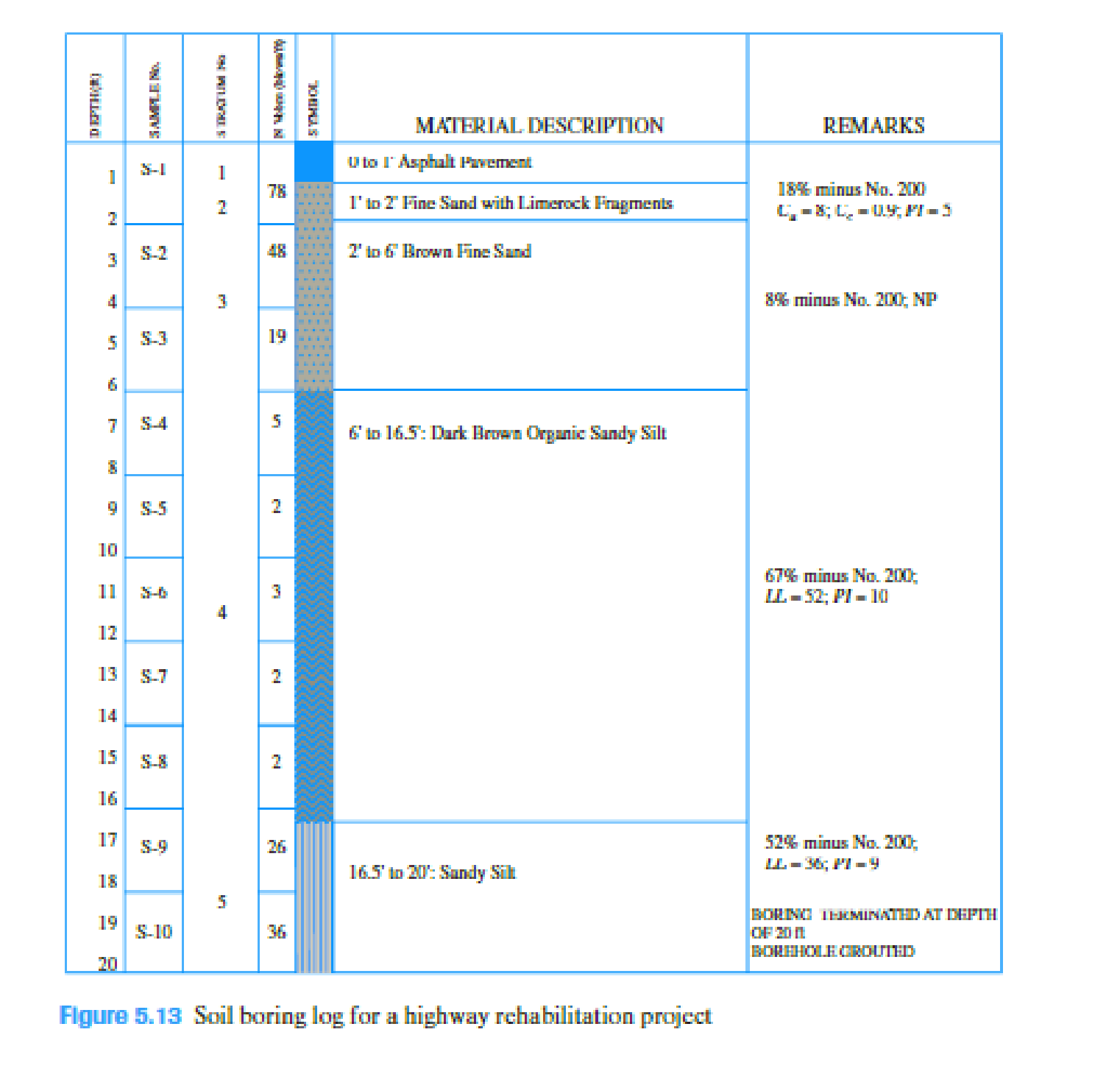Chapter 5, Problem 5.1CTPPrinciples of Geotechnical Enginee...

9th Edition
Braja M. Das + 1 other
ISBN: 9781305970939

Solutions

Chapter
SectionPrinciples of Geotechnical Enginee...

9th Edition
Braja M. Das + 1 other
ISBN: 9781305970939
Textbook Problem

The subsurface characteristics for a highway pavement rehabilitation project in the southeastern United States are shown in a “boring log” in Figure 5.13. The highway structure consists of the asphalt pavement underlain by four different soil strata up to a depth of 20 ft, after which the boring was terminated. Some data on the grain size and plasticity characteristics are also provided for each stratum. Perform the following tasks: 1. Determine the AASHTO soil classification and the group index (GI) for each layer. 2. Determine the “most probable” group symbols and group names for the various layers according to the Unified soil classification system. Use Table 5.3 and the soil characteristics given in the boring log.(a)

To determine

Find the AASHTO soil classification and the group index for each soil layer.

Explanation

Given information:

The subsurface characteristics for a highway pavement is given in the Table.

Calculation:

Consider stratum 2:

Consider 18% passing through No.200 sieve and plasticity index of soil is 5.

Find the group index (GI) using the formula:

GI=0.01(F15)(PI10)

Here, F is percent passing the No.200 sieve and PI is plasticity index.

Substitute 18 for F and 5 for PI.

GI=0.01(1815)(510)=0.150

Refer Table 4.1, “Classification of highway Subgrade Materials” in the textbook.

• Eighteen (18%) of soil passing through No.200 sieve, it falls under group

A-1-b because of the maximum percent of soil passing through No.200 is 25.

• For the soil group A-1-b, the maximum plasticity index is 6.

The soil is classified as A-1-b from the Table 4.1 by 18% of soil passing through No.200 sieve and has plasticity index of 5.

Therefore, the soil classification with group index for stratum 2 is A-1-b(0).

Consider stratum 3:

Consider 8% passing through No.200 sieve and plasticity index is NP (not possible).

Find the group index (GI) using the formula:

GI=0.01(F15)(PI10)

Here, F is percent passing the No.200 sieve and PI is plasticity index.

Plasticity index value is does not exist. Hence, the group index is considered as 0.

Refer Table 4.1, “Classification of highway Subgrade Materials” in the textbook.

• Eight (8%) of soil passing through No.200 sieve, it falls under group A-1-a, A-1-b, A-3, A-2-4, A-2-5, A-2-6 and A-2-7.
• For the soil group A-3, the plasticity index is NP.

The soil is classified as A-3 from the Table 4.1 by 8% of soil passing through No.200 sieve and has plasticity index is NP.

Therefore, the soil classification with group index for stratum 3 is A-3(0).

Consider stratum 4:

Consider 67% passing through No.200 sieve, the liquid limit is 52 and plasticity index of soil is 10.

Find the group index (GI) using the formula.

GI=(F35)[0.2+0.005(LL40)]+0.01(F15)(PI10)

Here, F is percent passing the No.200 sieve, LL is liquid limit, and PI is plasticity index.

Substitute 67 for F, 52 for LL, and 10 for PI.

GI=(6735)[0.2+0.005(5240)]+0

(b)

To determine

Find the most probable group symbols and group names for the various layers according to the Unified soil classification systems (USCS).

Still sussing out bartleby?

Check out a sample textbook solution.

See a sample solution

The Solution to Your Study Problems

Bartleby provides explanations to thousands of textbook problems written by our experts, many with advanced degrees!

Get Started